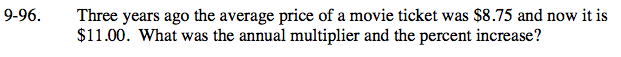### Home > CCA > Chapter 9 > Lesson 9.4.1 > Problem9-96

9-96.

Three years ago the average price of a movie ticket was $8.75 and now it is$11.00. What was the annual multiplier and the percent increase? Homework Help ✎$8.75(x)(x)(x) =$11.00

The annual multiplier is 1.075.
What is the percent increase?